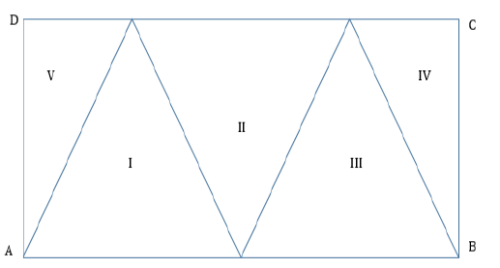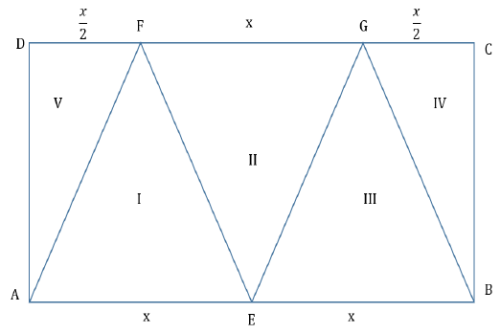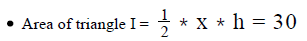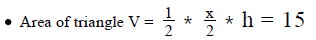In the figure given above, ABCD is a rectangl...

### Related TestIn the figure given above, ABCD is a rectangle that is divided into five triangular regions. If triangles I, II and III have equal area and triangles IV and V have equal area, what is the area of rectangle  ABCD?
(1) The area of triangle I is 30
(2) The area of triangle V is 15
• a)
Statement (1) ALONE is sufficient, but statement (2) alone is
• b)
Statement (2) ALONE is sufficient, but statement (1) alone is
• c)
BOTH statements (1) and (2) TOGETHER are sufficient to
• d)
EACH statement ALONE is sufficient to answer the question
• e)
Statements (1) and (2) TOGETHER are NOT sufficient to
problem are needed.PUNITH KAMINI SStep 1 & 2: Understand Question and Draw Inference
• Rectangle ABCD
• Triangles I, II and III have same areas
• As they lie between the same set of parallel lines, their height (h) would be equal
• So, their base would also be equal
• AB = AE + EB (but AE= EB), let’s assume AE=EB =x
• So, AB = 2x
• Similarly, base of triangle II = FG = x• Triangles IV and V have the same areas.
• As triangles IV and V lie between the same parallel line, their height (h) would be equal
• So, their bases would also be equal
• CD = AB = 2x
• DF + FG + GC = 2x
• 2DF + x= 2x
• DF = GC =
To Find: Area of rectangle ABCD = 2x * h
Step 3 : Analyze Statement 1 independent
(1) The area of triangle I is 30As we know the value of x*h, we can find the value of area of rectangle ABCD.
Step 4 : Analyze Statement 2 independent
(2) The area of triangle V is 15As we know the value of x*h, we can find the value of area of rectangle
ABCD.
Step 5: Analyze Both Statements Together (if needed)
As we have a unique answer from steps 3 and 4, this step is not required.View courses related to this question Explore GMAT courses
 Explore GMAT coursesView courses related to this question1 Crore+ students have signed up on EduRev. Have you?

• ### Directions: Each Data Sufficiency problem consists of a question and two st... more(Scan QR code)